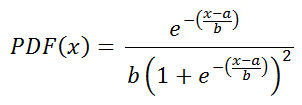# Logistic Distribution Random Number Generator

The logistic distribution resembles the normal distribution in that it is symmetric and has a central peak. Researchers sometimes use the logistic distribution instead of the Gaussian distribution because it has a simpler functional form that can be integrated more easily, which allows easier computation of probabilities.

The parameter a gives the center of the distribution, while the parameter b serves as a scaling factor.

 a =       b =       N = Decimal Precision integer tenths hundredths thousandths Display comma separated vertical

The density function of the logistic distribution with parameters a and b isThe mean, median, mode, variance, and skewness are simple functions of a and b:

mean = a
median = a
mode = a
variance = (π²/3)b²
skewness = 0